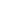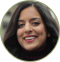Aditya Birla Sun Life Mutual Fund

MFGlossaryX For Extended Internal Rate of Return

Internal Rate of Return (IRR) is the interest rate at which the net present value of all the cash flows (both positive and negative) from a project or investment equal zero. IRR does not solve one problem and that is when the payments are at Irregular interval. In that case we use XIRR. So in a Spreadsheet we put the date and the value both signifies how much an investment usually offer and XIRR is a more powerful function in Excel to calculate annualized yield for a schedule of cash flows occurring at irregular periods.

My Financial Goal #1My Goal